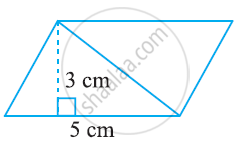Share

# Find the Area of the Following Parallelograms -2 - CBSE Class 7 - Mathematics

ConceptArea of a Parallelogram

#### Question

Find the area of the following parallelograms#### Solution

Area of parallelogram = Base × Height

Height= 3 cm

Base = 5 cm

Area of parallelogram = 5 × 3 = 15 cm2

Is there an error in this question or solution?

#### APPEARS IN

NCERT Solution for Mathematics for Class 7 (2018 to Current)
Chapter 11: Perimeter and Area
Ex. 11.20 | Q: 1.2 | Page no. 216
Solution Find the Area of the Following Parallelograms -2 Concept: Area of a Parallelogram.
S